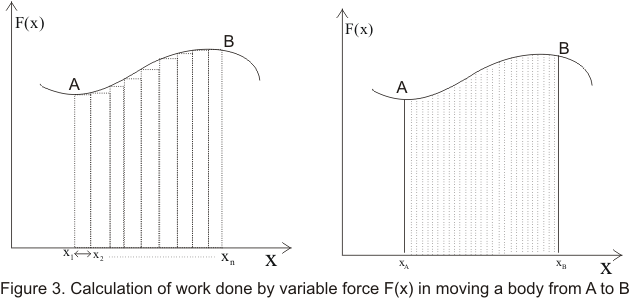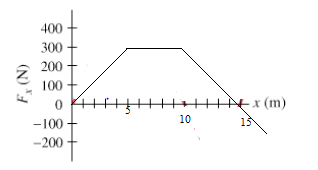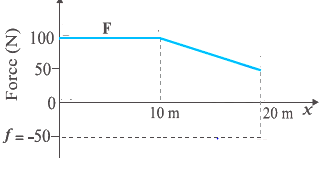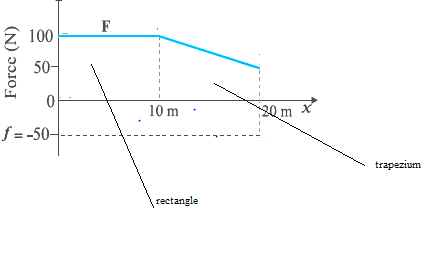# Work Done by the Variable force

## (3) Work done by variable force

• So far we have defined work done by a Force which is constant in both magnitude and direction.
• However, work can be done by forces that varies in magnitude and direction during the displacement of the body on which it acts.
• For simplicity consider the direction of force acting on the body to be along x-axis also consider the force F(x) is some known function of position x
• Now total displacement or path of the body can be decomposed into number of small intervals Δx such that with in each interval force F(x) can be considered to be approximately constant as shown below in the figure• Work done in moving the body from x1 to x2 is given by
ΔW=F(x1)Δx1 where Δx1=x2-x1
• Total work done in moving the body from point A to point B
W=F(x1)Δx1 +F(x2)Δx2 +F(x3)Δx3 .....+F(xn)Δxn
W=Σ F(xi)Δxi where i=1 to i=n           (4)
Where Σ is the symbol of summation
• Summation in equation 4 is equal to shaded area in figure 3(a) .More accuracy of results can be obtained by making these interval infinitesimally smaller
• We get the exact value of work done by making each interval so much small such that #916;x-> 0 which means curved path being decomposed into infinite number of line segment i.e
W= LimΔx-> 0Σ F(xi)Δxi           (5)
$W= \int_{x_a}^{x_b} fdx$ Where $\int_{x_a}^{x_b} fdx$ is the integral of F(x) w.r.t x between the limits xA and xB and integral can be evaluated using methods of calculus if F(x) of x is known
• Instead of x if the force also acts along y and z axis i.e direction of force keeps on changing then work done by such a force is given by

W=∫ F(r)dr with in the limits rA and rB           (6)
Where F(r)=F(x)i +F(y)j+ F(z)k
and dr=dxi +dyj+ dzk
Here F(x),F(y) and F(z) are rectangular components of the force along x ,y and z axis .Similarly dx,dy and dz are rectangular components of displacement along
x ,y and z axis
• Work done by the variable force along x-axis can also be calculated using the graphical way also. The area enclosed by the Force displacement gives the work done by the variable force

Example-1
A object of mass m= 2 kg is subjected by the variable force in x-direction. The force varies as per the below function
$F_x= (3 + .2 x) N$
Find the work done by the force in moving the object from x=0 to x= 5 m?
Solution
$W= \int_{x_a}^{x_b} fdx$
$W= \int_{0}^{5} (3 + .2x) dx = 17.5 J$

Example -2
A particle is acted by the force along x-axis which varies as given in below grapha. Find the work done from x=0 to x=5 m
b. Find the work done from x=5 to x=10 m
c. Find the work done from x=10 to x=15 m
Solution
Work done by will given by the area enclosed
a. work done from x=0 to x=5 m = $\frac {1}{2} \times 300 \times 5 = 750 N$
b. work done from x=5 to x=10 m = $300 \times 5 = 1500 N$
c. work done from x=10 to x=15 m = $\frac {1}{2} \times 300 \times 5 = 750 N$

Example- 3 A man pushes a trunk on a railway platform which has a rough surface. He applies a force of 100 N over a distance of 10 m. Thereafter, he gets progressively tired and his applied force reduces linearly with distance to 50 N. The total distance through which the trunk has been moved is 20 m. a. Plot the force applied by the man and the frictional force, which is 50 N versus displacement
b. Calculate the work done by the force applied by Man over 20 m.
c. Calculate the work done by the frictional forces over 20 m.
d. Calculate the net work done on trunk
Solution
The plot of the force applied by the man (F) and Frictional force (50 N) is given belowThe frictional force is constant and acts in the opposite direction to the applied force.So it is shown as negative in the graph

Now The work done by the man is given by the area enclosed by the Force F in the above graph
Work done= area of the rectangle + area of the trapezium$W= 100 \times 10 + \frac {1}{2} (100 + 50 ) \times 10$
= 1000 + 750
= 1750 J

Now The work done by the frictional force is given by the area enclosed by the friction force (-50 N) in the above graph
Work done= area of the rectangle
$W = -50 \times 20 = -1000 J$

Net work done= 1750 -1000 = 750 J